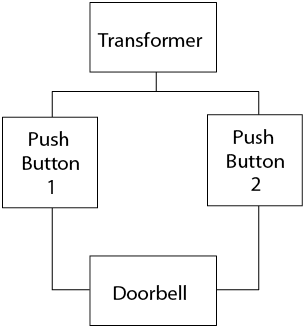# 6.2: Types of Electrical Diagrams

•• Camosun College
$$\newcommand{\vecs}{\overset { \rightharpoonup} {\mathbf{#1}} }$$ $$\newcommand{\vecd}{\overset{-\!-\!\rightharpoonup}{\vphantom{a}\smash {#1}}}$$$$\newcommand{\id}{\mathrm{id}}$$ $$\newcommand{\Span}{\mathrm{span}}$$ $$\newcommand{\kernel}{\mathrm{null}\,}$$ $$\newcommand{\range}{\mathrm{range}\,}$$ $$\newcommand{\RealPart}{\mathrm{Re}}$$ $$\newcommand{\ImaginaryPart}{\mathrm{Im}}$$ $$\newcommand{\Argument}{\mathrm{Arg}}$$ $$\newcommand{\norm}{\| #1 \|}$$ $$\newcommand{\inner}{\langle #1, #2 \rangle}$$ $$\newcommand{\Span}{\mathrm{span}}$$ $$\newcommand{\id}{\mathrm{id}}$$ $$\newcommand{\Span}{\mathrm{span}}$$ $$\newcommand{\kernel}{\mathrm{null}\,}$$ $$\newcommand{\range}{\mathrm{range}\,}$$ $$\newcommand{\RealPart}{\mathrm{Re}}$$ $$\newcommand{\ImaginaryPart}{\mathrm{Im}}$$ $$\newcommand{\Argument}{\mathrm{Arg}}$$ $$\newcommand{\norm}{\| #1 \|}$$ $$\newcommand{\inner}{\langle #1, #2 \rangle}$$ $$\newcommand{\Span}{\mathrm{span}}$$$$\newcommand{\AA}{\unicode[.8,0]{x212B}}$$

There are four basic types of electrical diagrams:

• schematic
• wiring
• block
• pictorial

## Schematic Diagrams

The schematic diagram (Figure $$\PageIndex{1}$$), often called a ladder diagram, is intended to be the simplest form of an electrical circuit. This diagram shows the circuit components on horizontal lines without regard to their physical location. It is used for troubleshooting because it is easy to understand the operation of the circuit. The loads are located on the far right of the diagram, and the controls for each load are located to the left. To understand the sequence of operation, the drawing is read from the upper left corner and then from left to right, and from top to bottom.Figure $$\PageIndex{1}$$: Schematic of a doorbell system (CC BY-NC-SA; BC Industry Training Authority)

## Wiring diagrams

The wiring diagram (Figure $$\PageIndex{2}$$) shows the relative layout of the circuit components using the appropriate symbols and the wire connections. Although a wiring diagram is the easiest to use for wiring an installation, it is sometimes difficult to understand circuit operation and is not as applicable for troubleshooting.Figure $$\PageIndex{2}$$: Wiring diagram (CC BY-NC-SA; BC Industry Training Authority)

## Block diagrams

The block diagram (Figure $$\PageIndex{3}$$), also called a functional block diagram, is used to describe the sequence of circuit operations. This diagram indicates by functional descriptions, showing which components must operate first in order to get a final outcome. They do not refer to specifics like device symbols or related wire connections.Figure $$\PageIndex{3}$$: Block diagram (CC BY-NC-SA; BC Industry Training Authority)

## Pictorial diagrams

A pictorial diagram (Figure $$\PageIndex{4}$$) shows the circuit components in more detail, as they really look, and indicates how the wiring is attached. These diagrams can be used to locate components in a complex system.Figure $$\PageIndex{4}$$: Pictorial diagram (CC BY-NC-SA; BC Industry Training Authority)Now complete the Learning Task Self-Test.